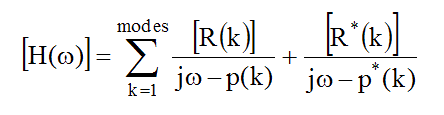# FRFs in Terms of Modal Parameters

FRF -based curve fitting assumes that any FRF calculated between two DOFs of a vibrating structure can be completely represented in terms of modal parameters.

## Partial Fraction Expansion of the FRF Matrix

The following parametric model is used to estimate experimental modal parameters by curve fitting FRF data.

• The FRF matrix is a summation of matrix pairs, each pair containing the contribution of a single mode (k).

• Each matrix pair for each mode (k) consists of a positive frequency term and a negative frequency (or complex conjugate) term.

• The unknown parameters of the parametric model are the modal frequency, damping & residue (mode shape component) for each mode of interest in the frequency band of the FRFs.

• The residues from a row or column of the FRF matrix are then saved as the mode shape for each mode.• [H(w)] - (n by n) FRF matrix.

• w -frequency variable     ( Hz or radians / second )

• p(k) - pole location for mode(k):  (p(k) = -s(k) + jw(k) )

• s(k) - damping decay constant for mode (k)   (in Hz or radians / second )

• w(k) - damped natural frequency for mode (k)     (in Hz or radians / second )

• [R(k)] - (n by n) Residue matrix for mode (k).

• n - number of DOFs of the MIMO model .

• modes - number of modes of interest on the structure.

• * - denotes the complex conjugate.

• j - denotes the imaginary axis in the complex plane.

## Local versus Global Curve Fitting

Local curve fitting is necessary when physical changes such as mass loading, temperature changes, or other effects cause the resonant frequencies & damping to change during the course of a modal test.

• The denominators of each term in the FRF matrix contain the same modal frequency & damping.

• Since all of the denominators are the same, frequency & damping can be estimated by curve fitting a single FRF, or all FRFs taken from the test article.

Generally speaking, modes are global properties of a structure.  Therefore, each mode has only one frequency & damping value, and one mode shape.

• During Local curve fitting, each FRF is curve fit and local frequency & damping estimates are obtained for each mode and each FRF.

• During Global curve fitting, multiple FRFs are curve fit and only one global frequency & damping estimate is obtained for each mode.

## Residue Matrix

A Residue represents the "strength" of a resonance.  That is, the stronger a resonance is relative to other resonances, the larger its residue will be.  In general, residues are complex numbers with magnitude & phase.

• In the partial fraction expansion equation, each mode (k) has a residue matrix [R(k)] associated with it.

• Each residue matrix has the same dimensions as the (n by n) MIMO model.

Residue units = ( FRF units ) x ( radians / second )
When one row or column of FRFs in the MIMO model is curve fit, the residues from the same row or column of the Residue matrix are estimated.

## Parameter Estimation (Curve Fitting)

During curve fitting, four modal parameters are estimated for each mode (k),
Frequency & damping, or the pole location p(k) = -s(k) + jw(k).
Magnitude & phase of the complex Residue R(k).